Sunday, October 25, 2020
Home > CBSE Class 12 > NCERT Solutions for Class 12 Maths Application of Derivatives Exercise 6.4

# NCERT Solutions for Class 12 Maths Application of Derivatives Exercise 6.4# NCERT Solutions for Class 12 Maths Application of Derivatives

Hi Students, Welcome to Amans Maths Blogs (AMB). In this post, you will get the NCERT Solutions for Class 12 Maths Application of Derivative Exercise 6.4.

NCERT Solutions for Class 12 Maths are not only the solutions of Maths exercise but it builds your foundation of other important subjects. Getting knowledge of depth concept of CBSE Class 12th Maths like Algebra, Calculus, Trigonometry, Coordinate Geometry help you to understand the concept of Physics and Physical Chemistry.

As we know that all the schools affiliated from CBSE follow the NCERT books for all subjects. You can check the CBSE NCERT Syllabus. Thus, NCERT Solutions helps the students to solve the exercise questions as given in NCERT Books

The PDF books of NCERT Solutions for Class 12 are the first step towards the learning and understanding the each sections of Maths, Physics, Chemistry, Biology as it all help in engineering medical entrance exams. To solve it, you just need to click on download links of NCERT solutions for class 12.

NCERT Solutions for class 12 is highly recommended by the experienced teacher for students who are going to appear in CBSE Class 12 and JEE Mains and Advanced and NEET level exams. Here You will get NCERT Solutions for Class 12 Maths Application of Derivative Exercise 6.2 of all questions given in NCERT textbooks of class 12 in details with step by step process.

CBSE Class 12th is an important school class in your life as you take some serious decision about your career. And out of all subjects, Maths is an important and core subjects. So CBSE NCERT Solutions for Class 12th Maths is major role in your exam preparation as it has detailed chapter wise solutions for all exercise. This NCERT Solutions can be downloaded in PDF file. The downloading link is given at last.

## NCERT Solutions for Class 12 Maths Application of Derivatives Exercise 6.4

NCERT Solutions for Class 12 Maths Application of Derivatives Exercise 6.4: Ques No 1.

Using differentials, find the approximate value of each of the following up to 3 places of decimal.

(i)NCERT Solutions:(ii)NCERT Solutions:(iii)NCERT Solutions: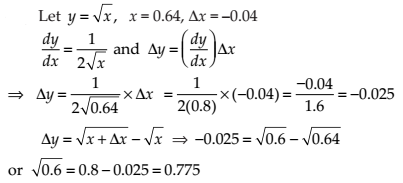(iv)NCERT Solutions: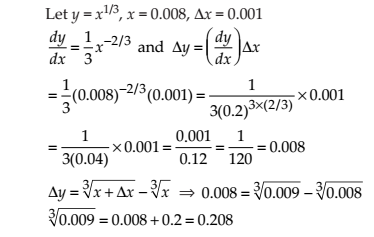(v)NCERT Solutions: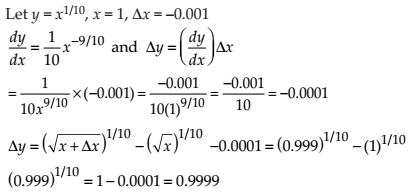(vi)NCERT Solutions: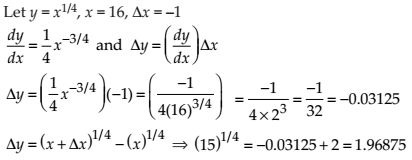(vii)NCERT Solutions: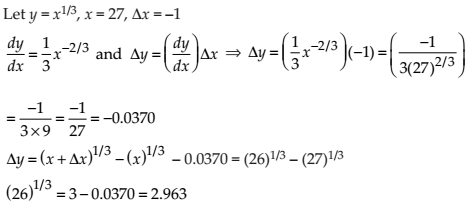(viii)NCERT Solutions:(ix)NCERT Solutions:(x)NCERT Solutions: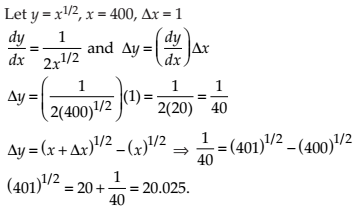(xi)NCERT Solutions: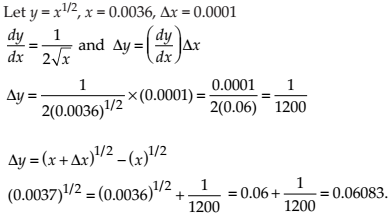(xii)NCERT Solutions: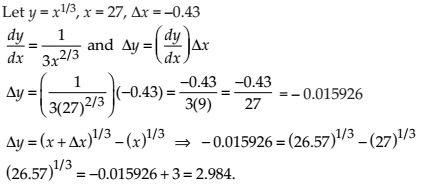(xiii)NCERT Solutions: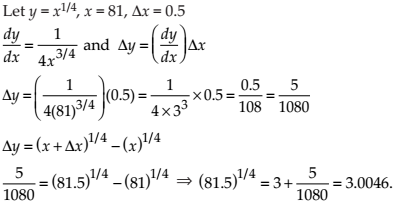(xiv)NCERT Solutions: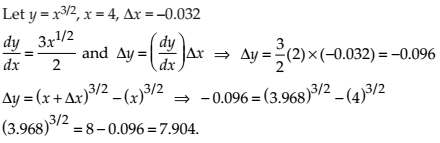(xv)NCERT Solutions:NCERT Solutions for Class 12 Maths Application of Derivatives Exercise 6.4: Ques No 2.

Find the approximate value of f(2.01), where f(x) = 4x2 + 5x + 2.

NCERT Solutions:NCERT Solutions for Class 12 Maths Application of Derivatives Exercise 6.4: Ques No 3.

Find the approximate value of f (5.001), where f (x) = x3 – 7x2 + 15.

NCERT Solutions: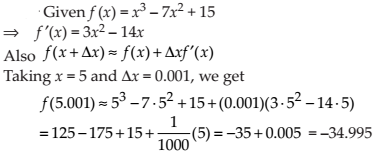NCERT Solutions for Class 12 Maths Application of Derivatives Exercise 6.4: Ques No 4.

Find the approximate change in the volume V of a cube of side x metres caused by increasing the side by 1%.

NCERT Solutions:NCERT Solutions for Class 12 Maths Application of Derivatives Exercise 6.4: Ques No 5.

Find the approximate change in the surface area of a cube of side x metres caused by decreasing the side by 1%.

NCERT Solutions: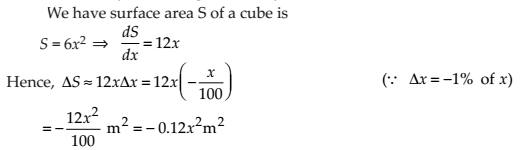NCERT Solutions for Class 12 Maths Application of Derivatives Exercise 6.4: Ques No 6.

If the radius of a sphere is measured as 7 m with an error of 0.02 m, then find the approximate error in calculating its volume.

NCERT Solutions: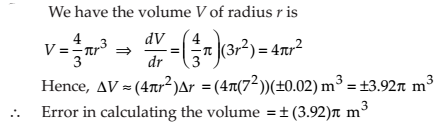NCERT Solutions for Class 12 Maths Application of Derivatives Exercise 6.4: Ques No 7.

If the radius of a sphere is measured as 9 m with an error of 0.03 m, then find the approximate error in calculating its surface area.

NCERT Solutions:NCERT Solutions for Class 12 Maths Application of Derivatives Exercise 6.4: Ques No 8.

If f(x) = 3x2 + 15x + 5, then the approximate value of f (3.02) is

(A) 47.66 (B) 57.66 (C) 67.66 (D) 77.66

NCERT Solutions: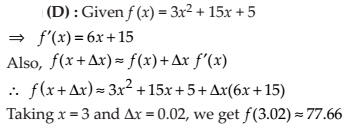NCERT Solutions for Class 12 Maths Application of Derivatives Exercise 6.4: Ques No 9.

The approximate change in the volume of a cube of side x metres caused by increasing the side by 3% is (A) 0.06 x3 m3 (B) 0.6 x3 m3 (C) 0.09 x3 m3 (D) 0.9 x3 m3

NCERT Solutions:error: Content is protected !!